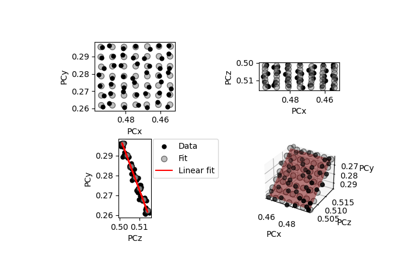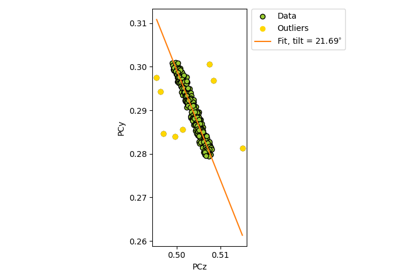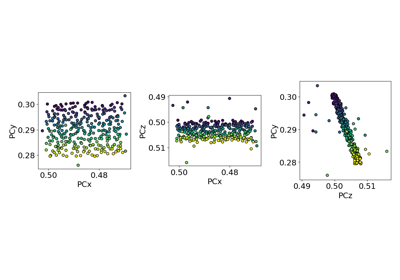# extrapolate_pc#

EBSDDetector.extrapolate_pc(pc_indices: , navigation_shape: tuple, step_sizes: tuple, shape: = None, px_size: = None, binning: = None, is_outlier: = None)[source]#

Return a new detector with projection centers (PCs) in a 2D map extrapolated from an average PC.

The average PC $$\bar{PC}$$ is calculated from pc, possibly excluding some PCs based on the is_outlier mask. The sample position having this PC, $$(\bar{x}, \bar{y})$$, is assumed to be the one obtained by averaging pc_indices. All other PCs $$(PC_x, PC_y, PC_z)$$ in positions $$(x, y)$$ are then extrapolated based on the following equations given in appendix A in :

$\begin{split}PC_x &= \bar{PC_x} + (\bar{x} - x) \cdot \Delta x / (\delta \cdot N_x \cdot b),\\ PC_y &= \bar{PC_y} + (\bar{y} - y) \cdot \Delta y \cdot \cos{\alpha} / (\delta \cdot N_y \cdot b),\\ PC_z &= \bar{PC_z} - (\bar{y} - y) \cdot \Delta y \cdot \sin{\alpha} / (\delta \cdot N_y \cdot b),\\\end{split}$

where $$(\Delta y, \Delta x)$$ are the vertical and horizontal step sizes, respectively, $$(N_y, N_x)$$ are the number of binned detector rows and columns, respectively, the angle $$\alpha = 90^{\circ} - \sigma + \theta$$, where $$\sigma$$ is the sample tilt and $$\theta$$ is the detector tilt, $$\delta$$ is the unbinned detector pixel size and $$b$$ is the binning factor.

Parameters:
pc_indices

2D map pixel coordinates (row, column) of each pc, possibly outside navigation_shape. Must be a flattened array of shape (2,) + navigation_size.

Shape of the output PC array (n rows, n columns).

step_sizes

Vertical and horizontal step sizes (dy, dx).

shape

Detector (signal) shape (n rows, n columns). If not given, (nrows, ncols) is used.

px_size

Unbinned detector pixel size. If not given, px_size is used.

binning

Detector binning factor. If not given, binning is used.

is_outlier

Boolean array with True for PCs to not include in the fit. If not given, all PCs are used. Must be of navigation_shape.

Returns:
new_detector

Detector with navigation_shape given by input navigation_shape.

## Examples using EBSDDetector.extrapolate_pc#Fit a plane to selected projection centers

Fit a plane to selected projection centersEstimate tilt about the detector x axis

Estimate tilt about the detector x axisEstimate tilts about the detector x and z axis

Estimate tilts about the detector x and z axis